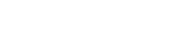# Project cost estimation template

Every project manager has to take care of the cost of a project. Use this to calculate it within Excel.

## Project cost estimation

Here's a simple method to create a project cost estimation template in Microsoft Excel:

1. Enter data into Excel regarding project cost. In the first row, create headings for the different categories of costs you want to include in your template. Some common categories include materials, labor, equipment, and overhead.2. Calculate the total of each stage by using the sum formula. Then drag it horizontally to get the sum of the other columns.3. Revise step two for stage 2 total.## Preparation of a project cost template

4. Now, add another column of total cost per unit, which includes all horizontal additions.5. Make two more rows below this table:6. Enter the number of units in the cell corresponding to the units to be produced. Then, multiply the units to be produced by the total cost per unit (both highlighted in red in the following screen shot). This is your total cost of production.This is how to estimate project costs in Excel. Save the template and use it for future project cost estimations.